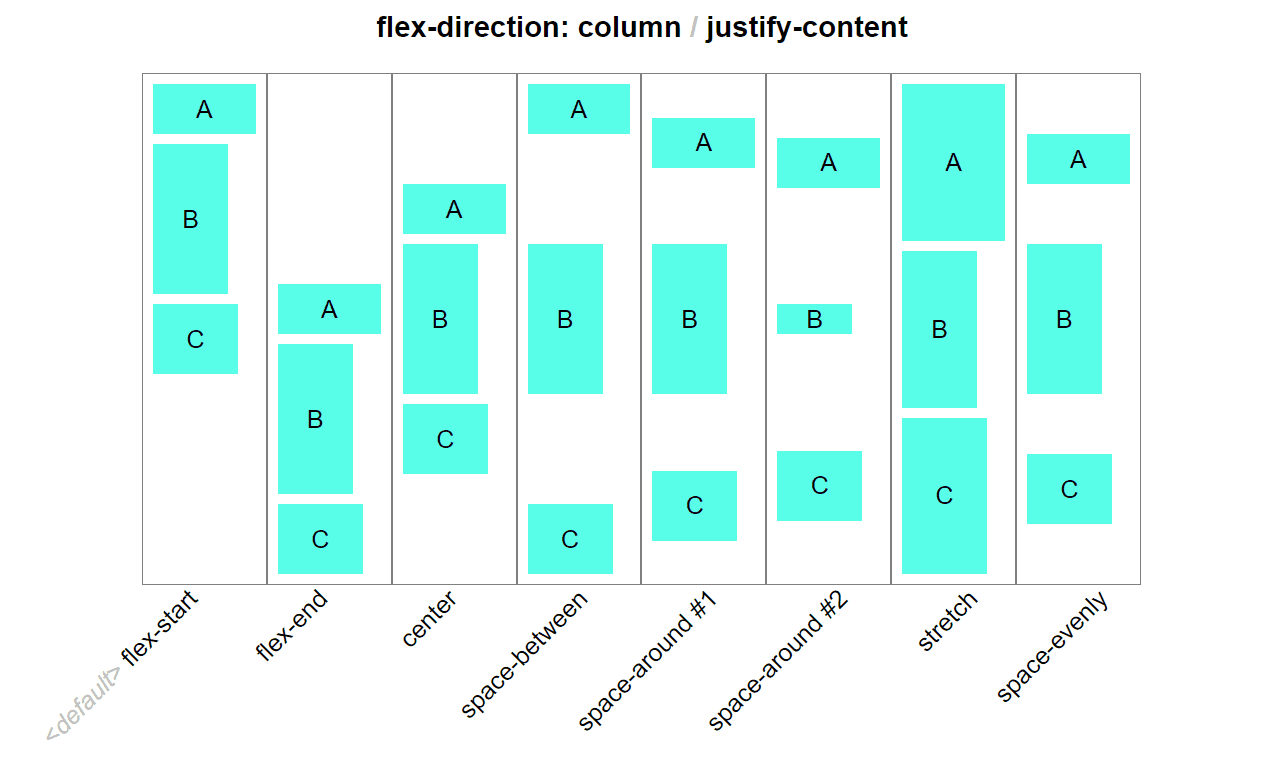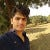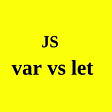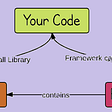# Difference between double equal and triple equal operators in JavaScript.

So difference between ==(Loose equality) and === (Strict equality check) operators is always a topic of discussion over the internet. Let’s find out how these two differ.

Loose Equality check or Double equals (==): The Double equals operator allows coercion(i.e conversion of one datatype to another) to happen during the comparison. The Double equals follows an algorithm while comparing two values. The algorithm is defined here https://www.ecma-international.org/ecma-262/#sec-abstract-equality-comparison .

We’ll go over the few points from this algorithm.

Point 1 : This point states that if the type of two variables are same then the strict equality comparsion is done and result is returned. So if the type of two operands are same, there is no difference between Double equals and triple equals.

Points 2 & 3: These points states the fact that null and undefined are equal to each other in loose equality comparsion. These two can be coerced to one another during the conversion.

Here double equals proves benefical if you don’t care whether a value is null or undefined, all you care is the value is an empty value.

Points 4 & 5 : These points defines that if any of the two operands(variable) is string. The string will be converted to number using the ToNumber() method and then the comparison will be done. The double equals favor numerical comparison.

Points 8 & 9 : These point deals with if any of the two operand is boolean then the boolean will be converted into the number using the ToNumber() method. The boolean value true will be converted to 1 while false will be converted to 0.

Points 10 & 11 : If any of the variable is an object then the object will be first passed through the ToPrimitive() method and then the comparison will be done.

If both the operand(variable) are objects, then true will be returned if both the objects are same objects(reference to the same object) otherwise false will be returned.

# Important points:

1. null and undefined are equal to each other.
2. -0 and +0 are equal.
3. -Infinity and + Infinity are not equal to each other.
4. NaN values are not equal to each other.

Strict Equality check or Triple equals (===): The triple equal doesn’t allow coercion to happen. It checks for the type of operands if two types are equal then the algorithm forwars to check the value of the operands otherwise it returns false.

null and undefined are treated as two seperate values.

# TL;DR

1. If the datatypes of the values involved are same, strict equality will be used.
2. Non-primitives are coerced to primitives if there is any.

--

--

--

## More from Nerd For Tech

NFT is an Educational Media House. Our mission is to bring the invaluable knowledge and experiences of experts from all over the world to the novice. To know more about us, visit https://www.nerdfortech.org/.

## DYK #1 [picture element]## Writing React like Vue 3## Implementing Promise.all, Promise.race, Promise.resolve and Promise.reject in Javascript## Tracing the origin of the Higher-order Components pattern## A JavaScript Crash Course — Introduction and Hello World## 2D Projectile Motion using Canvas and JS## Flexbox — The Animated TutorialWeb developer

## Redux Will Save React.js Headaches## let vs var : An underestimated difference in JS world## The Key Difference between a Framework and a Library in Javascript